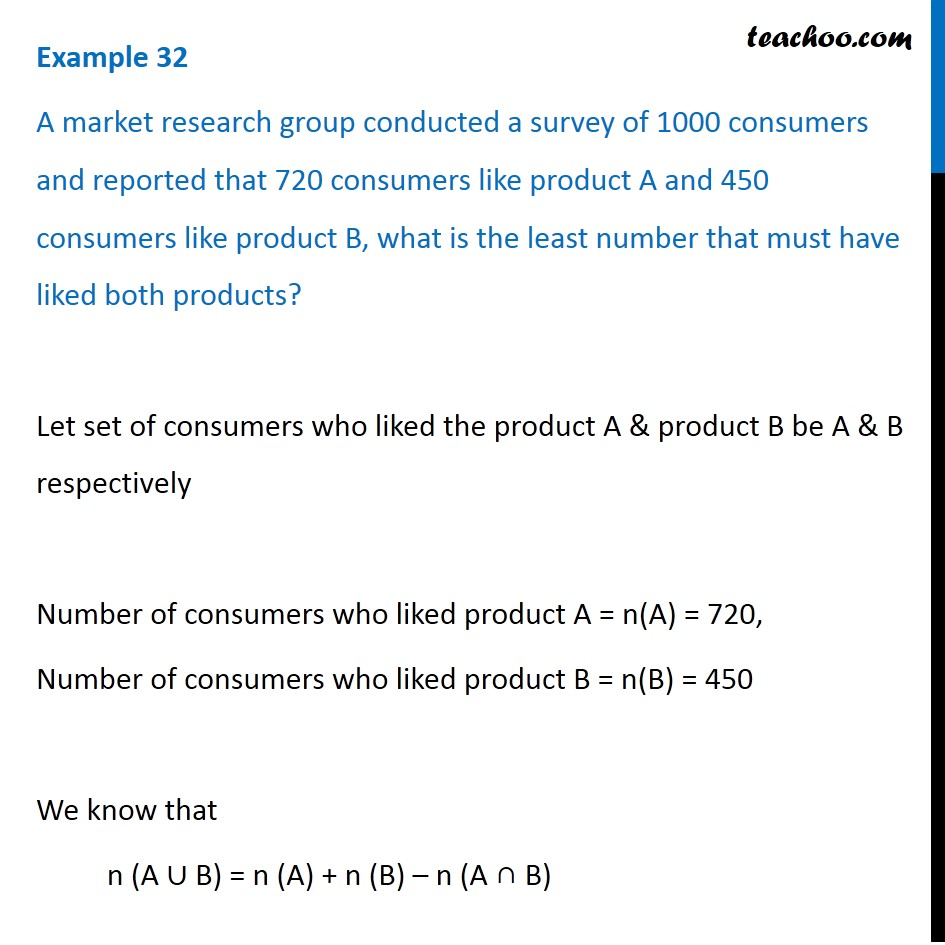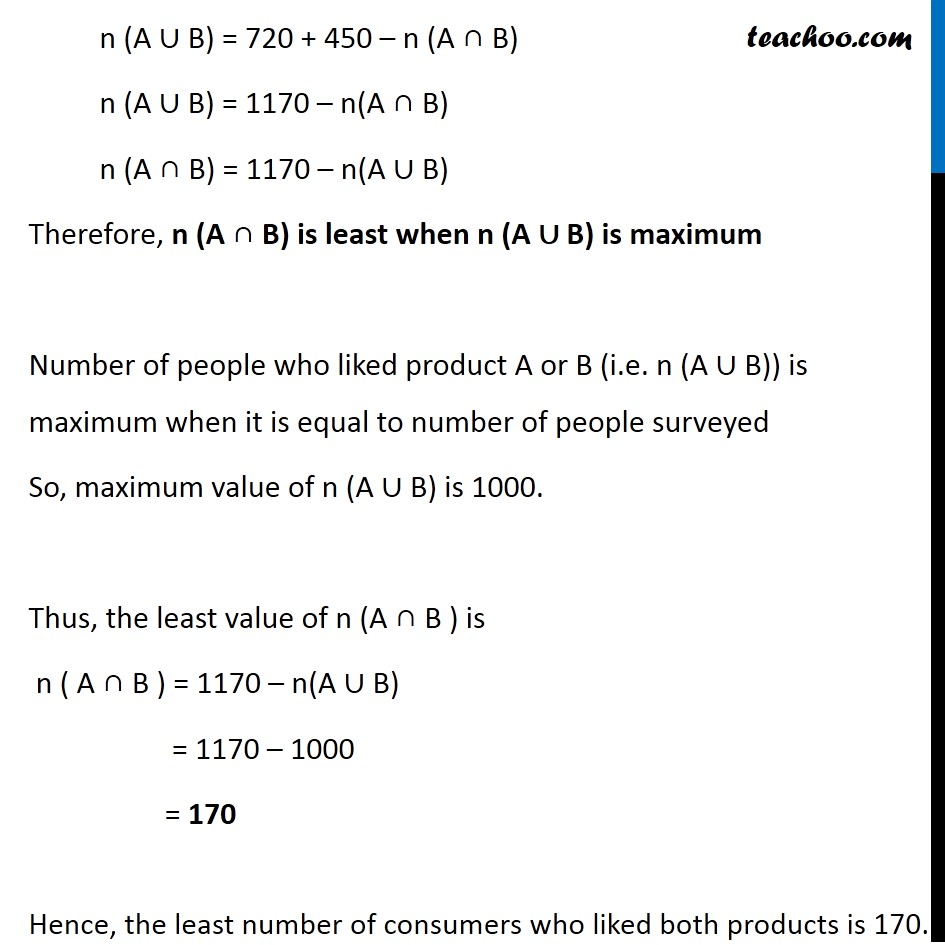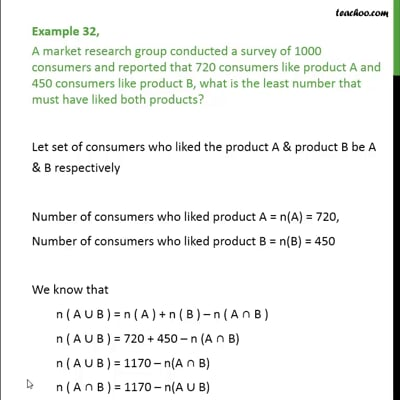Examples

Chapter 1 Class 11 Sets (Term 1)
Serial order wiseThis video is only available for Teachoo black users

### Transcript

Example 32 A market research group conducted a survey of 1000 consumers and reported that 720 consumers like product A and 450 consumers like product B, what is the least number that must have liked both products? Let set of consumers who liked the product A & product B be A & B respectively Number of consumers who liked product A = n(A) = 720, Number of consumers who liked product B = n(B) = 450 We know that n (A ∪ B) = n (A) + n (B) – n (A ∩ B) n (A ∪ B) = 720 + 450 – n (A ∩ B) n (A ∪ B) = 1170 – n(A ∩ B) n (A ∩ B) = 1170 – n(A ∪ B) Therefore, n (A ∩ B) is least when n (A ∪ B) is maximum Number of people who liked product A or B (i.e. n (A ∪ B)) is maximum when it is equal to number of people surveyed So, maximum value of n (A ∪ B) is 1000. Thus, the least value of n (A ∩ B ) is n ( A ∩ B ) = 1170 – n(A ∪ B) = 1170 – 1000 = 170 Hence, the least number of consumers who liked both products is 170. Example 33 Out of 500 car owners investigated, 400 owned car A and 200 owned car B, 50 owned both A and B cars. Is this data correct? Let the set of owners of car A & car B be A & B respectively Number of car A owned = n(A) = 400 Number of car B owned = n(B) = 200 Number of car A and car B owned = n(A ∩ B) = 50 We know that n (A ∪ B) = n (A) + n (B) – n (A ∩ B) = 200 + 400 – 50 = 550 So, number of people who owned either car A or car B = 550 But total number of car owners is only 500 (given in question) Number of people who own car A or car B should be less than total number of car owners investigated But, here 550 > 500 This is a contradiction. So, the given data is incorrect.